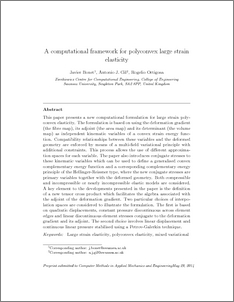# A computational framework for polyconvex large strain elasticity

, Gil, A.J. and Ortigosa, R. (2015) A computational framework for polyconvex large strain elasticity. Computer Methods in Applied Mechanics and Engineering, 283. pp. 1061-1094. ISSN 0045-7825 (Print), 1879-2138 (Online) (doi:https://doi.org/10.1016/j.cma.2014.10.002)Preview
PDF (Author's Pre-Print Version)
14088_BONET_Polyconvex_Large_Strain_Elasticity_2015.pdf - Submitted Version

## Abstract

This paper presents a novel computational formulation for large strain polyconvex elasticity. The formulation, based on the original ideas introduced by Schröder etal. (2011), introduces the deformation gradient (the fibre map), its adjoint (the area map) and its determinant (the volume map) as independent kinematic variables of a convex strain energy function. Compatibility relationships between these variables and the deformed geometry are enforced by means of a multi-field variational principle with additional constraints. This process allows the use of different approximation spaces for each variable. The paper extends the ideas presented in Schröder etal. (2011) by introducing conjugate stresses to these kinematic variables which can be used to define a generalised convex complementary energy function and a corresponding complementary energy principle of the Hellinger-Reissner type, where the new conjugate stresses are primary variables together with the deformed geometry. Both compressible and incompressible or nearly incompressible elastic models are considered. A key element to the developments presented in the paper is the new use of a tensor cross product, presented for the first time by de Boer (1982), page 76, which facilitates the algebra associated with the adjoint of the deformation gradient. For the numerical examples, quadratic interpolation of the displacements, piecewise linear interpolation of strain and stress fields and piecewise constant interpolation of the Jacobian and its stress conjugate are considered for compressible cases. In the case of incompressible materials two formulations are presented. First, continuous quadratic interpolation for the displacement together with piecewise constant interpolation for the pressure and second, linear continuous interpolation for both displacement and pressure stabilised via a Petrov-Galerkin technique.

Item Type: Article cited By 1 Complementary energy variational principle; Finite elements; Incompressible elasticity; Large strain elasticity; Mixed variational principle; Polyconvex elasticity T Technology > TA Engineering (General). Civil engineering (General) Vice-Chancellor's Group 10 May 2019 11:11 None GREAT a None GREAT 1 http://gala.gre.ac.uk/id/eprint/14088View Item